Algebra 1 1-9 Guided Practice: Patterns, Equations, and Graphs
starstarstarstarstarstarstarstarstarstar
by Matthew Richardson
| 18 Questions1
2
1
10
Solve It! The table shows the relationship between the number of kites in an arrangement and the total number of ribbons on the kites' tails.

Describe the pattern in the table.
2
10
How many kites could you make with 275 ribbons?

Enter only a number.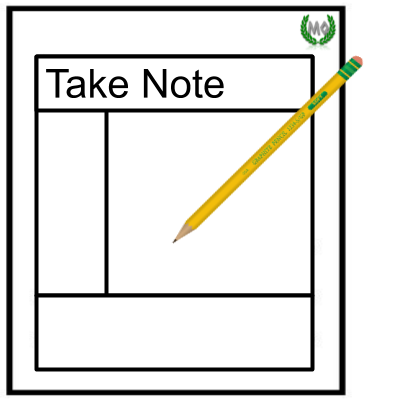3
4
3
10
Take Note: Define solution of an equation with two variables.
4
10
Take Note: Which of the following are solutions of the equation below?
Select all that apply.
(3,4)
(-1,5)
(2,3)
(-2,7)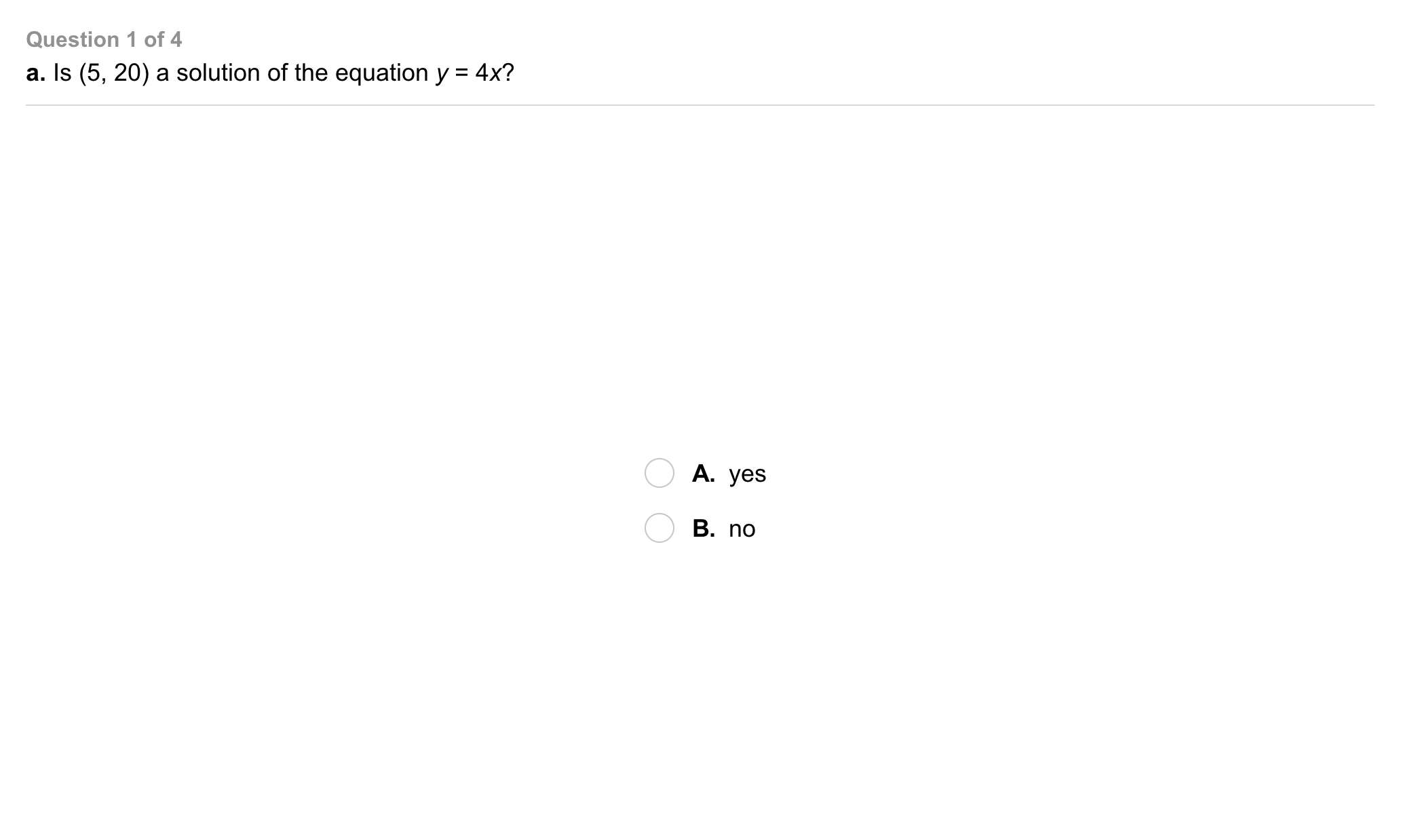5
5
5
Problem 1 Got It?
A
B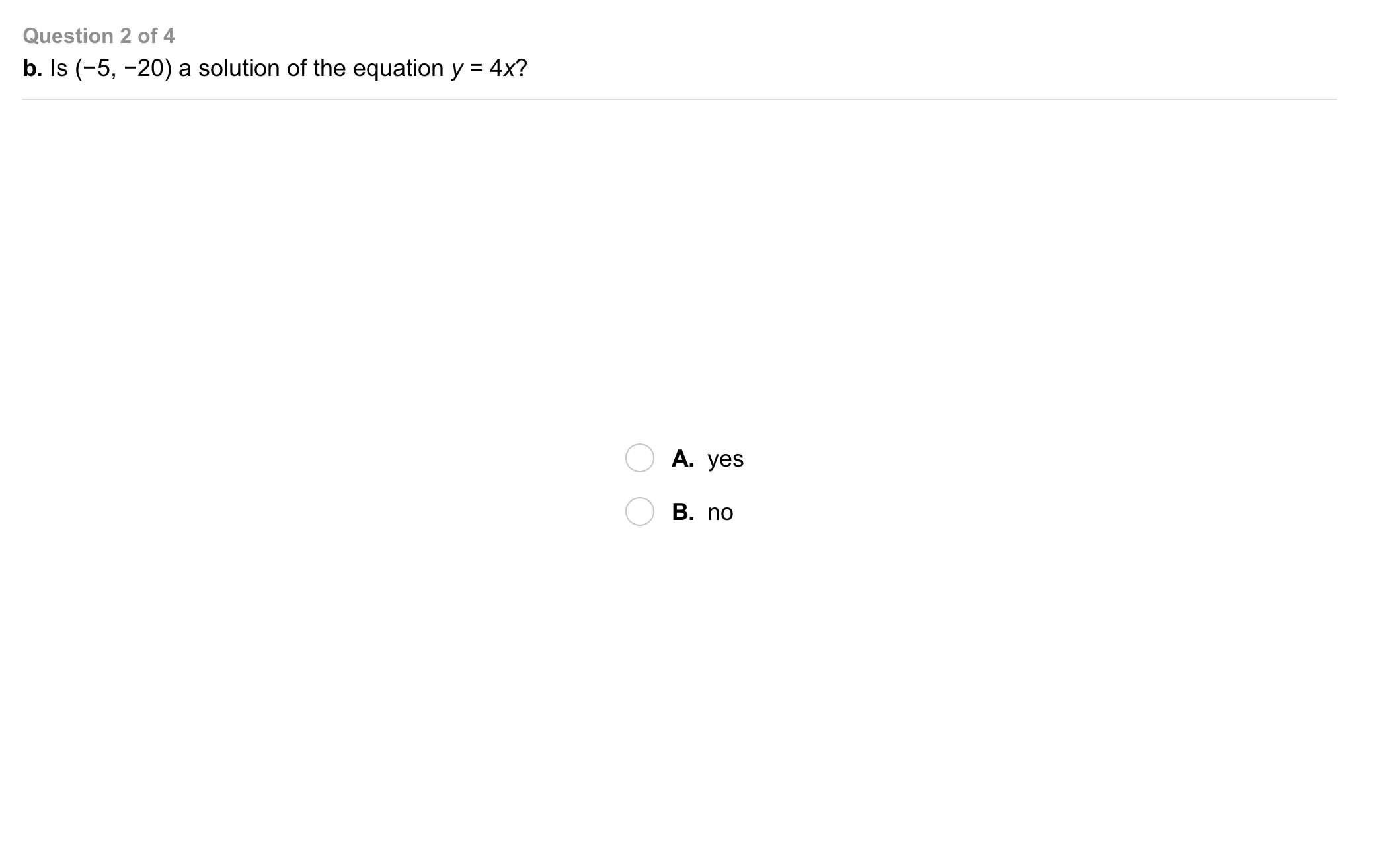6
6
5
Problem 1 Got It?
A
B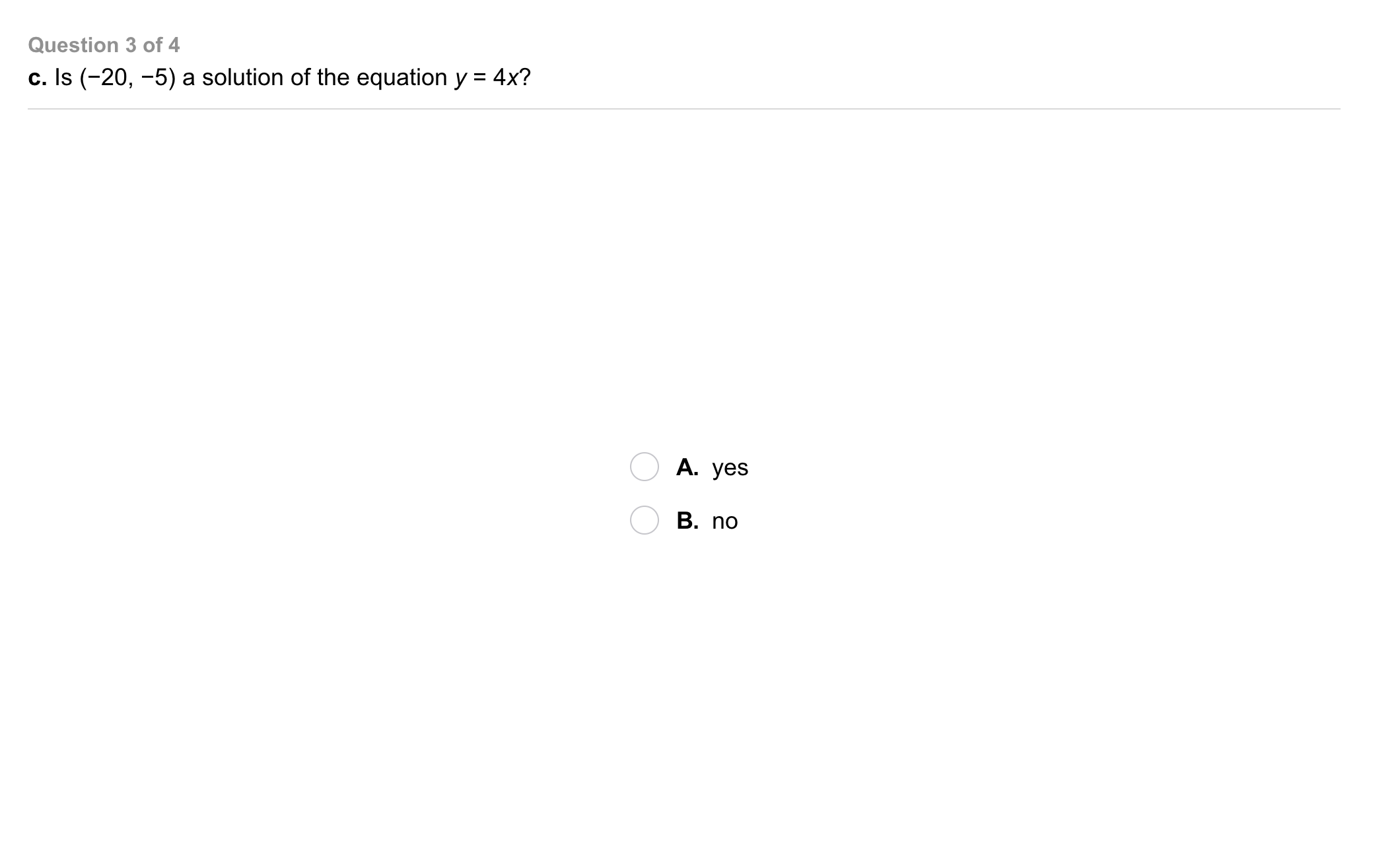7
7
5
Problem 1 Got It?
A
B8
8
5
Problem 1 Got It?
A
B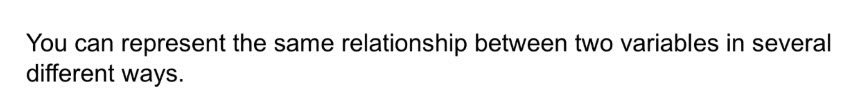9
10
Problem 2 Got It? Will runs 6 laps before Megan joins him at the track. They run together at the same pace. How can you represent the relationship between the number of laps Will runs and the number of laps Megan runs in a table?
10
10
Problem 2 Got It? Will runs 6 laps before Megan joins him at the track. They run together at the same pace. How can you represent the relationship between the number of laps Will runs and the number of laps Megan runs on a graph?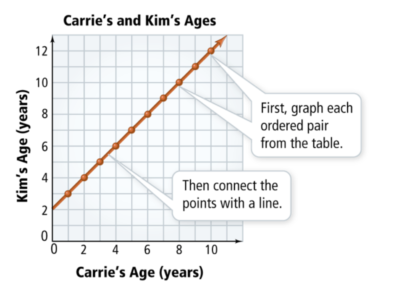11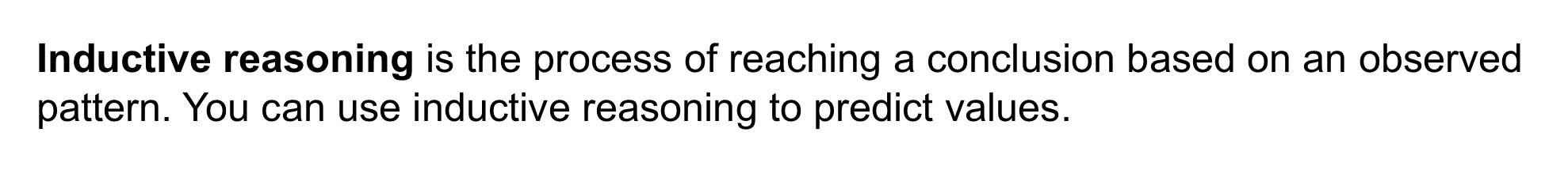12
13
12
10
Take Note: Define inductive reasoning.
13
10
Take Note: Use inductive reasoning to make a conclusion based on a pattern you have observed in real life. Describe the pattern and state your conclusion using complete sentences.14
15
14
10
Problem 3 Got It? Use the figure from problem 3 to make a table showing the number of orange tiles and the total number of tiles in each figure.
15
10
Problem 3 Got It? How many tiles in all will be in a figure with 24 orange tiles?

Enter only a number.16
17
16
10
Problem 3 Got It? Use the figure from problem 3 to make a table showing the number of blue tiles and the number of yellow tiles in each figure.
17
10
Problem 3 Got It? How many yellow tiles will be in a figure with 24 blue tiles?

Enter only a number.18
18
10
Take Note: Summarize the mathematical content of this lesson. What topics, ideas, and vocabulary were introduced?